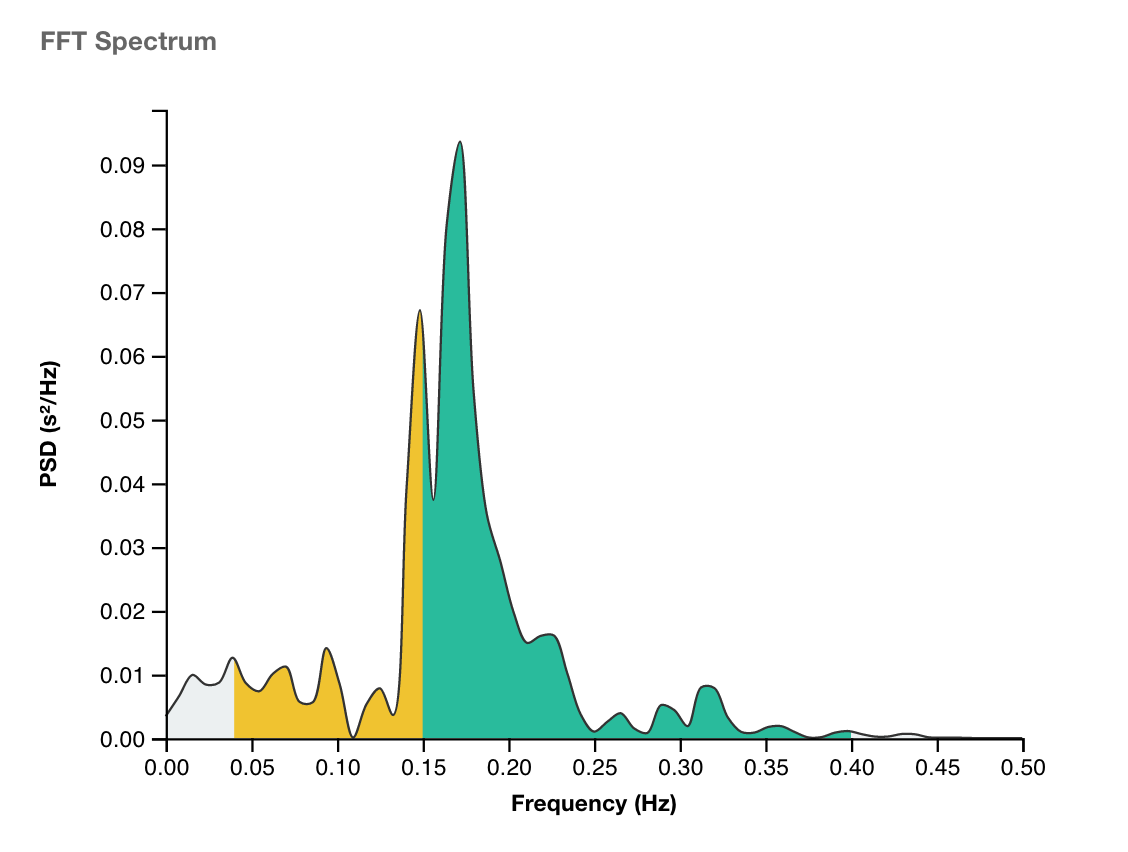# What are HRV Frequency Measurements (LF, HF, LF/HF)Heart Rate Variability (HRV) is a term that describes many metrics and analysis techniques, including Time Domain, Frequency Domain, and Non-Linear Analysis.

Frequency Domain Analysis is a complex analysis technique that shows how much of a signal lies within one or more frequency bands (ranges). With regards to Heart Rate Variability, research has identified certain frequency bands that tend to correlate with certain physiological phenomenon, such as Parasympathetic nervous system activity.

(Scroll to the bottom to understand the difference between units of measurement)

Common Frequency Domain HRV metrics include:

• High Frequency power (HF): frequency activity in the 0.15 - 0.40Hz range (green in the above chart)
• Low Frequency power (LF): frequency activity in the 0.04 - 0.15Hz range (yellow in the above chart)

Note that obtaining accurate lower frequency measurements requires longer reading times (minimum 4 minutes or more).

You may also have seen frequency values in Hertz (Hz) or milliseconds (ms) or milliseconds squared (ms 2).

Hertz (Hz) refers to the frequency band or the location on the frequency band. For example, there may be frequency activity at 0.4 Hz - or whichever band is the "peak" of the spectrum. This tells you where in the frequency spectrum you are referring to.

The actual activity in that band is typically expressed in terms of "power", which uses the units milliseconds squared (ms 2) for a particular Hertz (Hz) band. Think of it as "area under the curve".

For example, you might have 590 ms 2 of power in the 0.4hz frequency band (hypothetical example).